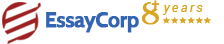WhatsApp
Struggling With Assignments?

We are here to help.

Chat with our expert writers on WhatsApp

for any kind of assignment queries.

Click to chat!
Struggling with Assignments? WhatsApp UsQuestion:

Using the 50-50 Rule, what is earned value for this project,Using the 0-100 Rule, what is earned value for this project,Note the discrepancy of earned value figures when using the 50-50 Rule and 0-100,Why is there a discrepancy, Which Rule should we use? Explain your rationale.

1. The Earned value for this project is explained here
While using the 50-50 technique, PV=EV. So, the values are=

The cumulative EV for all tasks = EV1+EV2+EV3+EV4+EV5+EV6=19,600.

2. The earned value for the project is same using the 0-100 rule.

3. There is a discrepancy in the values, which are seen in after application of 50-50 and 0-100 rule. The difference is due to the following reasonIn the 0-100 technique, no EV is credited until a certain task is complete, so this approach is good for short discrete tasks. For the 50-50 rule, half value of the EV is credited once the tasks are started and other half on completion. This is generally done when the tasks are long, and have multiple reporting periods. This helps in keeping a tab on the projects for managers. For this project, the 0-100 rule is the best. As it has also been stated that the project is complete, this rule is considered best for this project.

4. The formula to be used for Schedule Variance is as followsSV=EV-PV Since, the project is on schedule, the SV (Schedule Variance) is zero. This means, as stated in the question; the project has been completed on time.

5. The SPI calculated as followsSPI=EV/PV Since the project is on schedule, the value is one . It means that the project is on schedule.

6. The cost variance for the project is as followsCV=
EV- AC

7. The CPI is calculated as followsCPI=
EV/AC

8. The can be calculated as follows
In first step,
ETC = (BAC - EV) / CPI,
The BAC value

HIRE EXPERT
Email ID *
Subject
Pages